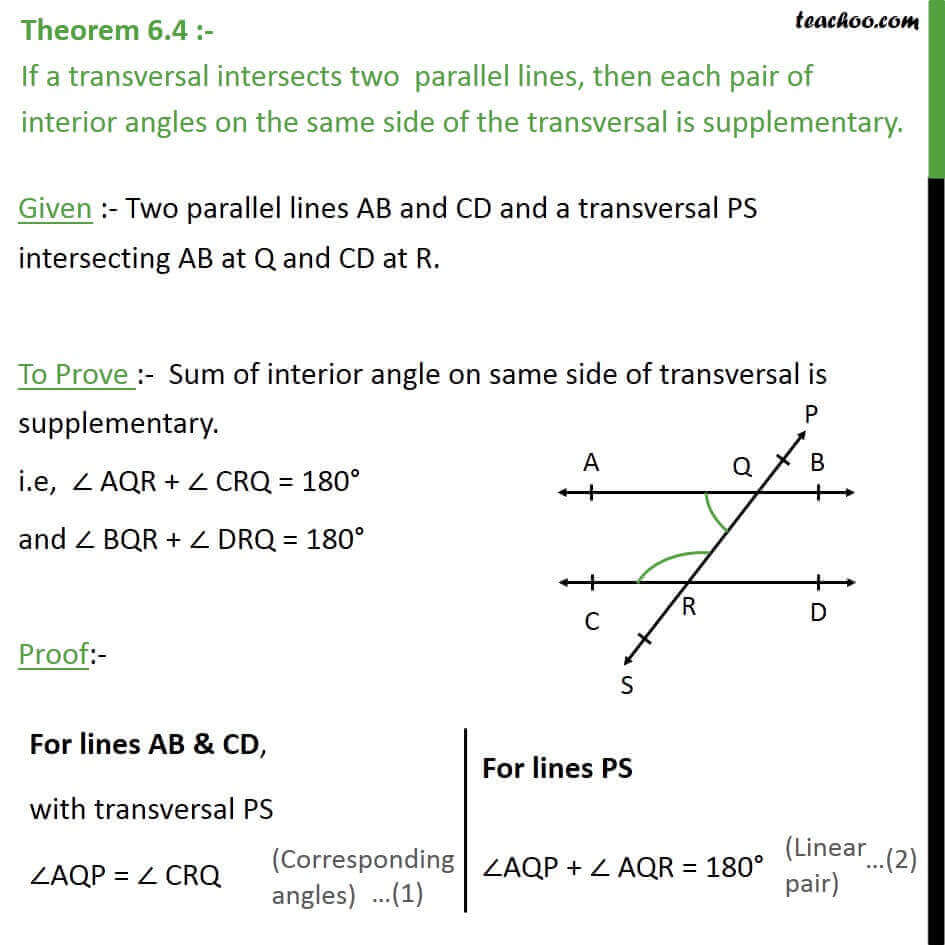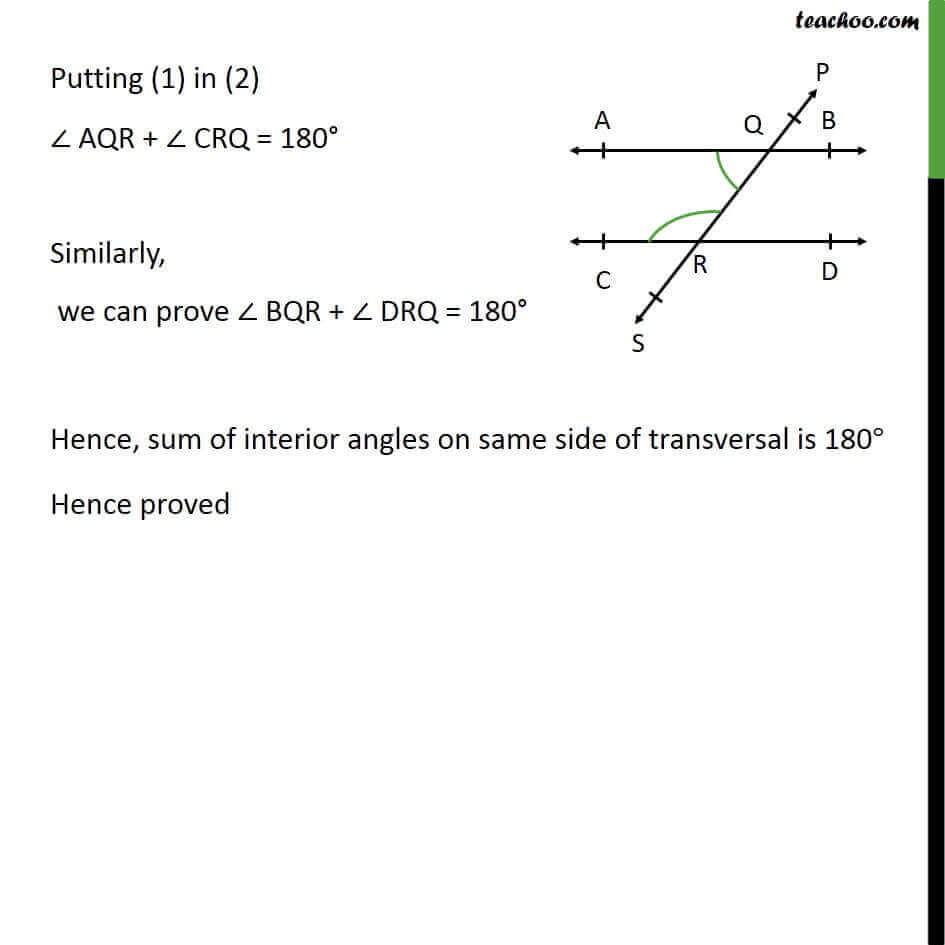Theorems

Chapter 6 Class 9 Lines and Angles
Serial order wiseGet live Maths 1-on-1 Classs - Class 6 to 12

### Transcript

Theorem 6.4 :- If a transversal intersects two parallel lines, then each pair of interior angles on the same side of the transversal is supplementary. Given :- Two parallel lines AB and CD and a transversal PS intersecting AB at Q and CD at R. To Prove :- Sum of interior angle on same side of transversal is supplementary. i.e, ∠ AQR + ∠ CRQ = 180° and ∠ BQR + ∠ DRQ = 180° Proof:- Putting (1) in (2) ∠ AQR + ∠ CRQ = 180° Similarly, we can prove ∠ BQR + ∠ DRQ = 180° Hence, sum of interior angles on same side of transversal is 180° Hence proved